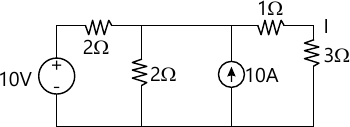MORE IN Basic Electricals & Electronics Engg.
RGPV First Year Engineering (Set A) (Semester 1)
Basic Electricals & Electronics Engg.
December 2014
Total marks: --
Total time: --
INSTRUCTIONS
(1) Assume appropriate data and state your reasons
(2) Marks are given to the right of every question
(3) Draw neat diagrams wherever necessary

Solve any one question from Q1 & Q1 (e)
1 (a) Write the major difference between:
i) Ideal voltage source and practical voltage source
ii) Dependent and independent sources
2 M
1 (b) Define :
i) Impedance and
ii) Phase sequence in a.c. circuit.
2 M
1 (c) Explain with units and
i) Real power
ii) Reactive power
iii) Apparent power in a.c. circuit.
3 M
1 (d) Derive the relation for conversion for star and delta connection.
7 M
1 (e) State Thevenin?s theorem. Determine the current through a 3Ω resistor branch in the circuit using Thevenin?s theorem.14 M

Solve any one question from Q2 & Q2 (e)
2 (a) Define magnetic leakage and fringing.
2 M
2 (b) Give the reason of eddy current loss in transformer core.
2 M
2 (c) Define voltage regulation and efficiency of a transformer. Give the formula also.
3 M
2 (d) Draw the complete phasor diagram of a single phase transformer for an inductive load. Also write the notations used for all voltages and currents used in the phasor diagram.
7 M
2 (e) The results of tests performed on 1ϕ, 20 KVA, 2200/220 volt, 50 Hz. Transformer are as follows-
O.C. test : 220 V, 4.2 A, 148 W
S.C. test : 86 V, 10.5 A, 360 W.
Determine:
The regulation and efficiency at 0.8 p.f. lagging at full load.
14 M

Solve any one question from Q3 & Q3 (e)
3 (a) Write the necessity and material used for the following in a d.c. machine
i) Commutator
ii) Brush
2 M
3 (b) Why synchronous machine is called as synchronous. Define synchronous speed.
2 M
3 (c) Classify self excited D.C. motor.
3 M
3 (d) Derive the e.m.f. equation of a 3 phase Induction Motor.
7 M
3 (e) Draw and explain the complete Torque-slip characteristics of 3 phase induction motor.
14 M

Solve any one question from Q4 & Q4 (e)
4 (a) State and explain De Morgan?s theorem.
2 M
4 (b) Simplify the Boolean function Z = AB + AC + BC and therefore design the logic circuit using AND or OR logic gates.
2 M
3 M
4 (d) Explain number systems used in digital electronics.
7 M
4 (e) Explain in detail J-K flip flop.
14 M

Solve any one question from Q5 & Q5 (e)
5 (a) Define ideal diode and practical diode.
2 M
5 (b) Differentiate between conductor, semiconductor and insulator with example.
2 M
5 (c) Draw and explain the V-I, characteristic of diode.
3 M
5 (d) Draw the connection diagram and explain the use and working of CE transistor configuration.
7 M
5 (e) Explain the working of BJT. Discuss DC biasing of BJT.
14 M

More question papers from Basic Electricals & Electronics Engg.